# Euler deconvolution of synthetic magnetic data¶

Euler deconvolution of potential fields is a widely used interpretation technique. This is, in no small part, due to its simplicity and ease of implementation and use. This IPython notebook will apply standard Euler deconvolution on synthetic total field magnetic anomaly data in an attempt to highlight some common misconceptions in the interpretation of the results.

We use the Euler deconvolution implemented in the open-source Python library Fatiando a Terra. The library also provides routines for plotting, forward modeling, calculating derivatives through FFT (required for Euler deconvolution) and more.

Euler deconvolution is based on Euler's homogeneity equation,

$$(x_i - x_0)\dfrac{\partial f_i}{\partial x} + (y_i - y_0)\dfrac{\partial f_i}{\partial y} + (z_i - z_0)\dfrac{\partial f_i}{\partial z} = \eta (b - f_i),$$

where $(x_i, y_i, z_i)$ are the coordinates of observation $f_i$ (the total field anomaly), $(x_0, y_0, z_0)$ is a point on the source (where that point is depends on the nature of the source), $b$ is a constant base level (shift in the data), and $\eta$ is called the structural index (SI) and is related to the nature of the source.

Given the observed total field anomaly $f_i$, its derivatives, and the structural index, we can solve the above equation for the unknown point $(x_0, y_0, z_0)$ and base level $b$. This is implemented as the class Classic from the euler module of Fatiando a Terra.

However, this is only valid if there is only one source. When there are multiple sources, the most common approach is to select a small window over the data and solve using the observations that fall inside the window. The window is then moved and another solution is found. Thus, we end up with a set of solutions. This is called a moving window scheme and is implemented in class MovingWindow in the euler module.

A drawback of this approach is the sheer number of solutions generated, many of which are spurious. Thus, the solutions have to be filtered so that only consistent solutions are kept. How to best perform this filtering is an active field of research and will not be covered here. The MovingWindow class implements a simple filter that keeps only a percentage of the solutions, ranked by the average uncertainty of the estimate. This is an approach similar to that adopted by Beiki and Pedersen (2010).

Returning to the subject of the structural index, it is a number that characterizes the decay of the potential field with distance. The structural indexes of some ideal sources (sphere, cylinder, vertical dike, etc) have been calculated (e.g., Reid et al., 1990). However, most, if not all, geologic sources cannot be properly approximated by such ideal sources. There are methods that have been developed to estimate the structural index during Euler deconvolution. However, that is outside the scope of our study and will not be covered here.

We will show an application of Euler deconvolution using the moving window scheme on synthetic total field magnetic anomaly data. This application will illustrate how the deconvolution results behave for non-ideal sources. We will use the results obtained to highlight how the output of Euler deconvolution should and, most importantly, should not be interpreted.

The first step is to load (import) the required libraries.

In :
%matplotlib inline
import numpy as np
import json
import cPickle as pickle
from IPython.display import Image
from fatiando.gravmag.euler import Classic, ExpandingWindow, MovingWindow
from fatiando.gravmag import fourier, sphere, polyprism
from fatiando.mesher import PolygonalPrism, Sphere
from fatiando.vis import mpl, myv
from fatiando import utils
import fatiando
print("Using Fatiando a Terra version {0}".format((fatiando.version)))
mpl.rc('font', size=9)

Using Fatiando a Terra version 0.2


Now, we load the synthetic data and accompanying metadata using modules json and numpy.

In :
x, y, z, tf = np.loadtxt('data/synthetic_data.txt', unpack=True)

In :
metadata

Out:
{u'area': [5000, 25000, 5000, 25000],
u'bounds': [0, 30000, 0, 30000, 0, 5000],
u'dec': 30,
u'inc': -15,
u'shape': [100, 100]}

Module mpl of Fatiando a Terra interfaces with the 2D plotting library matplotlib. We can use it to generate a filled contour plot of the data. Note that the x coordinates (array x) are North-South and y (array y) are East-West.

In :
mpl.figure(figsize=(3.33, 2.7))
mpl.axis('scaled')
mpl.contourf(y, x, tf, shape, 60, cmap=mpl.cm.RdBu_r)
mpl.colorbar().set_label('nT')
mpl.m2km()
mpl.xlabel('East (km)')
mpl.ylabel('North (km)')
mpl.savefig('fig/data.png', dpi=600)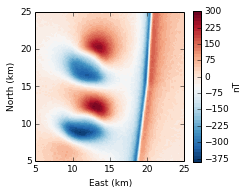The polygonal prism model used to generate the data is stored in a Python pickle file.

In :
with open('data/model.pickle') as f:
dike, sill, batholith = model


We can use module myv to interface with 3D plotting library Mayavi and render the model. Notice that the model is composed of 3 different structures: a slightly crooked vertical dike, an irregular sill and a structure resembling an intrusive body, like a batholith.

In :
scene = myv.figure(size=(1000, 600))
myv.polyprisms([dike], linewidth=1, color=(0, 0, 1))
myv.polyprisms([sill], linewidth=1, color=(0, 1, 0))
myv.polyprisms([batholith], linewidth=1, color=(1, 0, 0))
ax = myv.axes(myv.outline(bounds), ranges=[b*0.001 for b in bounds], nlabels=3, fmt='%.0f')
ax.axes.x_label, ax.axes.y_label, ax.axes.z_label = 'x', 'y', 'z'
ax.axes.font_factor = 2
myv.wall_north(bounds)
myv.wall_bottom(bounds)
scene.scene.camera.position = [-37282.427190894407, -35382.676458226633, -36760.719551179718]
scene.scene.camera.focal_point = [13286.06353429469, 14199.525065081265, 5611.7451127001987]
scene.scene.camera.view_angle = 19.2
scene.scene.camera.view_up = [0.37050322249789591, 0.35546774432369316, -0.85812006436401433]
scene.scene.camera.clipping_range = [42859.55137481264, 132512.66650687021]
scene.scene.camera.compute_view_plane_normal()
scene.scene.render()
myv.savefig('fig/model.png')
myv.show()
Image(filename='fig/model.png')

Out: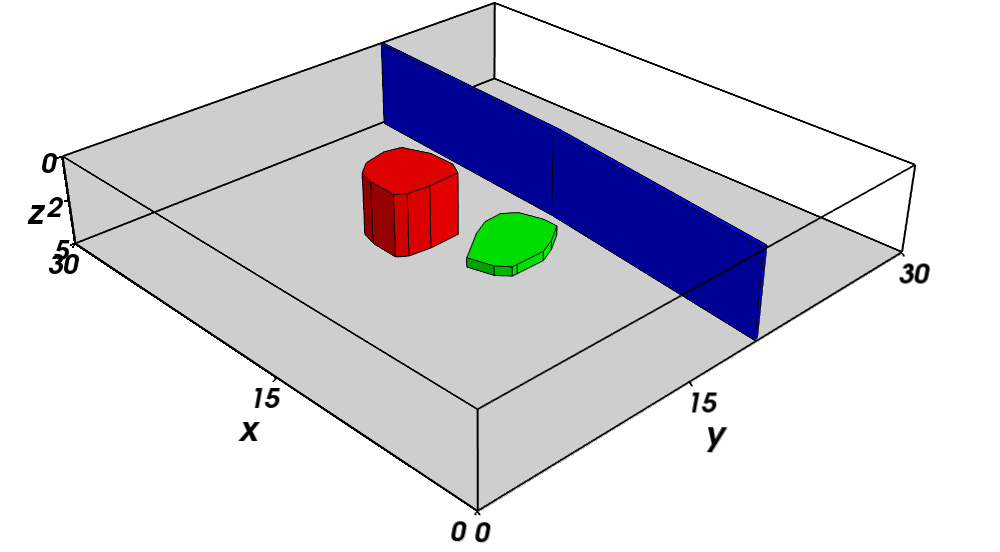Lets have a look at some of the properties of the model:

In :
print('Dike:')
print(' top (m): {}'.format(dike.z1))
print(' bottom (m): {}'.format(dike.z2))
print(' magnetization (SI): {}'.format(dike.props['magnetization']))

print('Sill:')
print(' top (m): {}'.format(sill.z1))
print(' bottom (m): {}'.format(sill.z2))
print(' magnetization (SI): {}'.format(sill.props['magnetization']))

print('Batholith:')
print(' top (m): {}'.format(batholith.z1))
print(' bottom (m): {}'.format(batholith.z2))
print(' magnetization (SI): {}'.format(batholith.props['magnetization']))

Dike:
top (m): 0.0
bottom (m): 5000.0
magnetization (SI): 10
Sill:
top (m): 1000.0
bottom (m): 1500.0
magnetization (SI): 10
Batholith:
top (m): 500.0
bottom (m): 4000.0
magnetization (SI): 2


## Structural index¶

The structural index (SI) characterizes the nature of the source of the anomaly. It will vary depending on the potential field, hence, the SI for a type of source will not be same for gravity and magnetic fields. SI values have been calculated for ideal sources. After Reid et al. (1990), the SIs for the magnetic case are

Source SI
Dike 1
Sill 1
Sphere 3
Vertical pipe 2

We'll assume that the SI values that best represent our model are: 1 for the dike and sill, 3 for the batholith.

## Calculating derivatives¶

Euler deconvolution requires the derivatives of the total-field anomaly. They could be directly measured (if possible) or calculated numerically. The important thing is that the derivatives need to be calculated/measured at the same observation points $(x_i, y_i, z_i)$ as the data. We will use module fourier of Fatiando a Terra to calculate derivatives using the FFT. Note that this requires the total field anomaly to be in the Système International units and on a regular grid. Output derivatives will be in units of T/m.

In :
tf_si = utils.nt2si(tf)
xderiv = fourier.derivx(x, y, tf_si, shape)
yderiv = fourier.derivy(x, y, tf_si, shape)
zderiv = fourier.derivz(x, y, tf_si, shape)

In :
mpl.figure(figsize=(10, 4))
levels = 30
cbargs = dict(orientation='horizontal')

ax = mpl.subplot(1, 3, 1)
mpl.axis('scaled')
mpl.title('x derivative')
mpl.contourf(y, x, xderiv, shape, levels, cmap=mpl.cm.RdBu_r)
mpl.colorbar(**cbargs).set_label('T/m')
mpl.m2km()
mpl.xlabel('East (km)')
mpl.ylabel('North (km)')

ax = mpl.subplot(1, 3, 2)
mpl.axis('scaled')
mpl.title('y derivative')
mpl.contourf(y, x, yderiv, shape, levels, cmap=mpl.cm.RdBu_r)
mpl.colorbar(**cbargs).set_label('T/m')
mpl.m2km()
mpl.xlabel('East (km)')
mpl.ylabel('North (km)')

ax = mpl.subplot(1, 3, 3)
mpl.axis('scaled')
mpl.title('z derivative')
mpl.contourf(y, x, zderiv, shape, levels, cmap=mpl.cm.RdBu_r)
cb = mpl.colorbar(**cbargs).set_label('T/m')
mpl.m2km()
mpl.xlabel('East (km)')
mpl.ylabel('North (km)')

Out:
<matplotlib.text.Text at 0x7711ed0>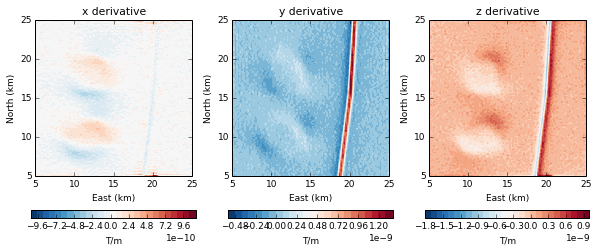## Euler deconvolution¶

Now we have all that we need to perform an Euler deconvolution with a moving-window scheme. We will use varying structural indexes (SI) and moving window sizes to illustrate the dependence of the results on these parameters.

First, we'll use a 1 km window and vary the structural index from 1 to 3. We'll store the results in a Python list for later use. The parameter keep=0.3 tells the moving window scheme to keep only the best 30% of solutions using the criterion of Beiki and Pedersen (2010). Note that we have to pass the total field anomaly and the derivatives in Système International units.

In :
euler_s1 = [MovingWindow(Classic(x, y, z, tf_si, xderiv, yderiv, zderiv, i),
windows=(50, 50), size=(1000, 1000), keep=0.3).fit()
for i in [1, 2, 3]]


Now we do the same for a 3 km window.

In :
euler_s3 = [MovingWindow(Classic(x, y, z, tf_si, xderiv, yderiv, zderiv, i),
windows=(50, 50), size=(3000, 3000), keep=0.3).fit()
for i in [1, 2, 3]]


For the sake of completeness, let's run on a 5 km window as well.

In :
euler_s5 = [MovingWindow(Classic(x, y, z, tf_si, xderiv, yderiv, zderiv, i),
windows=(50, 50), size=(5000, 5000), keep=0.3).fit()
for i in [1, 2, 3]]


The typical way that deconvolution results are show is using a scatter map with color (or point size) representing the depth (z coordinate). We'll make 6 plots, one for each combination of window size and structural index. While we plot, we'll also filter out unrealistic results that lie above the data.

In :
# Get the maximum value of z that we got from all runs.
# We'll use this to set the limits of the color bars
maxz = max(p for euler in [euler_s1, euler_s3] for e in euler for p in e.estimate_)
labels = ['(a)', '(b)', '(c)', '(d)', '(e)', '(f)']
fig = mpl.figure(figsize=(7, 4.8))
for j, euler in enumerate([euler_s1, euler_s3]):
for i, e in enumerate(euler):
xc, yc, zc = e.estimate_.T
xc, yc, zc = xc[zc > 0], yc[zc > 0], zc[zc > 0]
ax = mpl.subplot(2, 3, 3*j + i + 1)
mpl.axis('scaled')
mpl.text(6000, 23000, labels[3*j + i], fontsize=12, fontweight='bold')
mpl.title('SI={0} | Window={1:.0f} km'.format(e.euler.model['structural_index'], e.size*0.001))
#for body in model:
#    mpl.polygon(body, xy2ne=True, style='-b', linewidth=1.5)
plot = mpl.scatter(yc, xc, s=30, c=zc*0.001, cmap=mpl.cm.RdYlGn_r, vmin=0, vmax=maxz*0.001)
mpl.set_area(area)
mpl.m2km()
if i in [1, 2, 4, 5]:
ax.set_yticklabels([])
if j == 1:
mpl.xlabel('East (km)')
ax.set_xticklabels(['{:.0f}'.format(t*0.001) for t in ax.get_xticks()[:-1]])
if j in :
ax.set_xticklabels([])
if i in [0, 3]:
mpl.ylabel('North (km)')
fig.colorbar(plot, cax=mpl.axes([0.91, 0.09, 0.03, 0.85]), orientation='vertical').set_label('Depth (km)')
mpl.savefig('fig/euler-solutions-low.png')
mpl.savefig('fig/euler-solutions.png', dpi=600)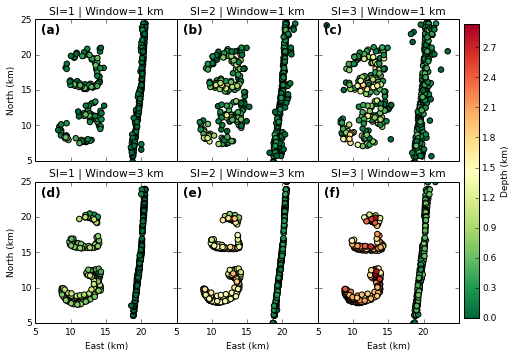In :
# Make the figure for the 5km window separately because it won't go in the tutorial
maxz = max(p for euler in [euler_s5] for e in euler for p in e.estimate_)
fig = mpl.figure(figsize=(12, 3.5))
for i, e in enumerate(euler_s5):
xc, yc, zc = e.estimate_.T
xc, yc, zc = xc[zc > 0], yc[zc > 0], zc[zc > 0]
ax = mpl.subplot(1, 3, i + 1)
mpl.axis('scaled')
mpl.title('SI={0} | Window={1:.0f} km'.format(e.euler.model['structural_index'], e.size*0.001))
plot = mpl.scatter(yc, xc, s=30, c=zc*0.001, cmap=mpl.cm.RdYlGn_r, vmin=0, vmax=maxz*0.001)
mpl.set_area(area)
mpl.m2km()
mpl.xlabel('East (km)')
mpl.ylabel('North (km)')
fig.colorbar(plot, cax=mpl.axes([0.91, 0.09, 0.03, 0.85]), orientation='vertical').set_label('Depth (km)')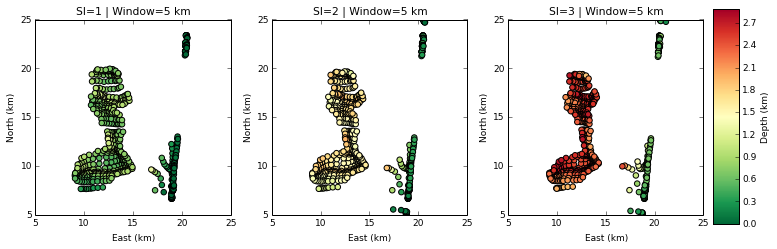Notice how changing the window size influences the spread of the solutions and can affect our interpretation. For example, for a 1 km window and structural index 3, we cannot tell apart the sill from the intrusive body. The solutions get even more tangled when using a 5 km window.

Varying the structural index will give different depths for the solutions. A general trend is that, the larger the structural index, the deeper the solutions.

Most importantly, we call attention to the fact that the Euler deconvolution solutions do not represent the three-dimensional shape of the sources. This is even more evident when we view the solutions in 3D along with the original model. For convenience, we'll plot only the solutions for a 3 km window and structural index 3.

In :
scene = myv.figure(size=(1000, 600))
myv.polyprisms(model, opacity=0.3, linewidth=2)
myv.points([e for e in euler_s3.estimate_ if e > 0])
ax = myv.axes(myv.outline(bounds), ranges=[b*0.001 for b in bounds], nlabels=3, fmt='%.0f')
ax.axes.x_label, ax.axes.y_label, ax.axes.z_label = 'x', 'y', 'z'
ax.axes.font_factor = 2
myv.wall_north(bounds)
myv.wall_bottom(bounds)
scene.scene.camera.position = [-10753.852296021096, -59325.007533753123, -22266.66194804628]
scene.scene.camera.focal_point = [16722.015964135739, 13784.075401721391, 4529.0411289607291]
scene.scene.camera.view_angle = 19.2
scene.scene.camera.view_up = [0.12859903242950413, 0.29830909042254711, -0.94576634293543571]
scene.scene.camera.clipping_range = [43526.915602369831, 131500.63847030781]
scene.scene.camera.compute_view_plane_normal()
scene.scene.render()
myv.savefig('fig/euler-solutions-3d.png')
scene.scene.camera.position = [61783.513706029873, -51534.690503972124, -18750.237814442207]
scene.scene.camera.focal_point = [14324.659515610345, 12669.434351699714, 2305.8434486142896]
scene.scene.camera.view_angle = 5.033164800000001
scene.scene.camera.view_up = [-0.14301336238889156, 0.21138117409951956, -0.96688426267807892]
scene.scene.camera.clipping_range = [41498.787075197979, 137750.00692720726]
scene.scene.camera.compute_view_plane_normal()
scene.scene.render()
myv.savefig('fig/euler-solutions-3d-zoom.png')
myv.show()
Image(filename='fig/euler-solutions-3d.png')

Out: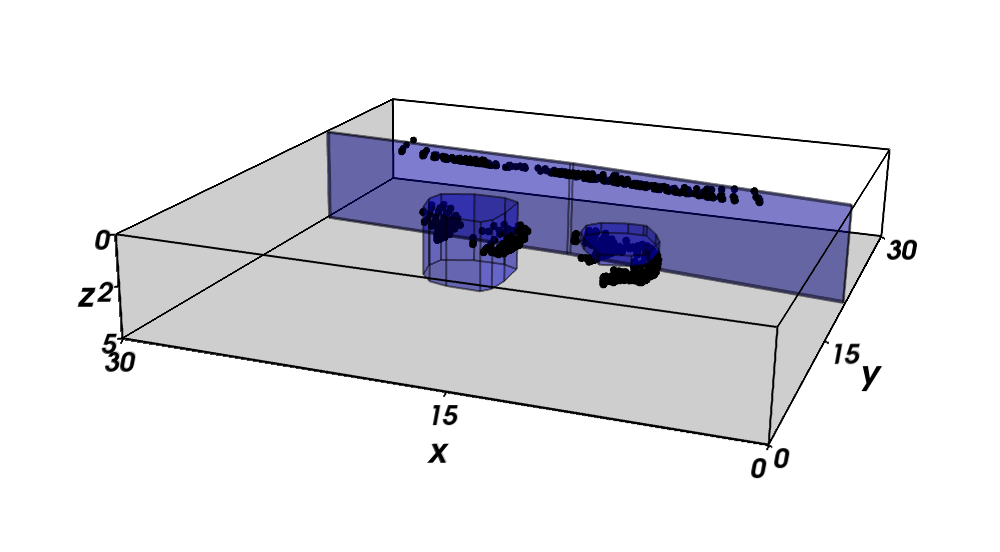In :
Image(filename='fig/euler-solutions-3d-zoom.png')

Out: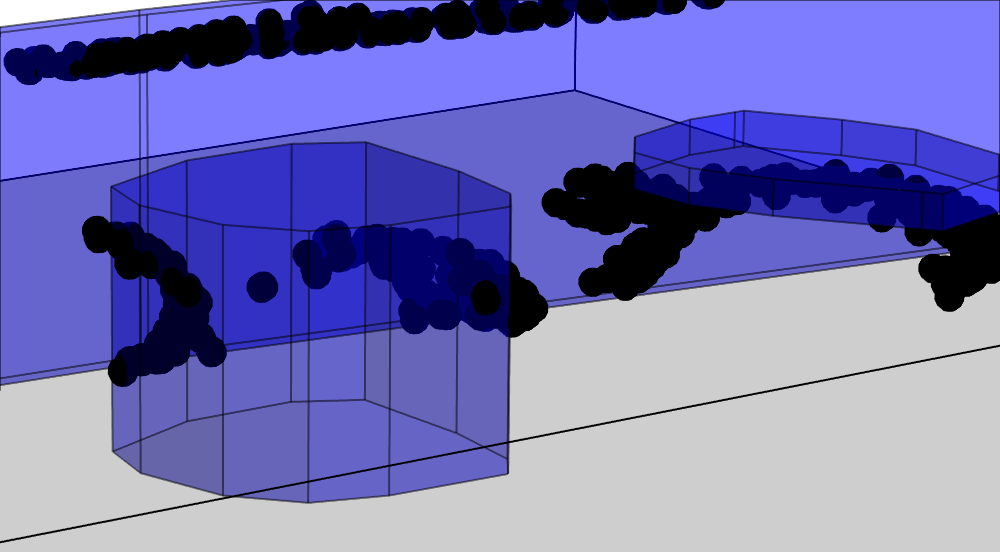Notice how the sources for the intrusive body and the sill make arch-like structures that have no relation to the actual forms of the sources. This behaviour is expected and it has been show that Euler deconvolution solutions have a bias that depends on the moving-window scheme and selection criterion (Silva et al., 2001).

Now, how much of this spread is due to our model not being properly represented by a sphere? One way to test that is to replace our batholith model with a sphere, forward model new data, and perform the Euler deconvolution on this new data.

## Solutions for a sphere¶

First, lets create a sphere that resembles our batholith model.

In :
ideal_batholith = Sphere(18000, 12000, 2250, radius=2200, props=batholith.props)
ideal_batholith.center = [ideal_batholith.x, ideal_batholith.y, ideal_batholith.z]

In :
scene = myv.figure(size=(800, 300))
myv.polyprisms([batholith], opacity=0.5, linewidth=2)
ax = myv.axes(myv.outline(bounds), ranges=[b*0.001 for b in bounds], nlabels=3, fmt='%.0f')
ax.axes.x_label, ax.axes.y_label, ax.axes.z_label = 'x', 'y', 'z'
ax.axes.font_factor = 2
myv.wall_north(bounds)
myv.wall_bottom(bounds)
scene.scene.camera.position = [-15337.932586926319, -59396.608310493262, -7022.645217992851]
scene.scene.camera.focal_point = [17482.870070805882, 15380.030101355769, 4903.8791935470208]
scene.scene.camera.view_angle = 9.830400000000001
scene.scene.camera.view_up = [0.064932345527732721, 0.12931668910473115, -0.98947510550961171]
scene.scene.camera.clipping_range = [40404.996563304114, 131956.0403000123]
scene.scene.camera.compute_view_plane_normal()
scene.scene.render()
myv.savefig('tmp/sphere-batholith.png')
myv.show()
Image(filename='tmp/sphere-batholith.png')

Out: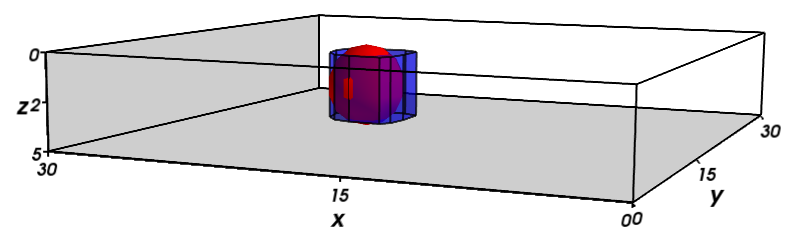Now we can forward model the new total field anomaly data from our model. To make it realistic, lets add 5 nT Gaussian noise (the same that was added to the original data).

In :
tf_sphere = utils.nt2si(
utils.contaminate(
sphere.tf(x, y, z, [ideal_batholith], inc, dec) + polyprism.tf(x, y, z, [dike, sill], inc, dec),
5))


Plot the two data sets side-by-side for comparison.

In :
mpl.figure(figsize=(10, 3.5))
mpl.subplot(1, 2, 1)
mpl.title('Polygonal prism')
mpl.axis('scaled')
mpl.contourf(y, x, tf_si, shape, 60, cmap=mpl.cm.RdBu_r)
mpl.colorbar().set_label('T')
mpl.m2km()
mpl.xlabel('East (km)')
mpl.ylabel('North (km)')
mpl.subplot(1, 2, 2)
mpl.title('Sphere')
mpl.axis('scaled')
mpl.contourf(y, x, tf_sphere, shape, 60, cmap=mpl.cm.RdBu_r)
mpl.colorbar().set_label('T')
mpl.m2km()
mpl.xlabel('East (km)')
mpl.ylabel('North (km)')

Out:
<matplotlib.text.Text at 0x9a66450>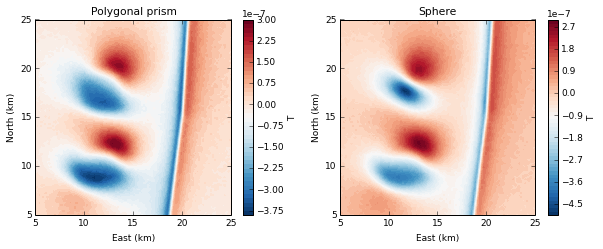It is clear that the data is not exactly the same. The anomaly caused by the sphere is less spread out and its negative lobe has a larger value. However, it is this difference in the anomaly that makes the use of a structural index 3 for the batholith model not very precise.

Now we can run the Euler deconvolution on the data set with the sphere and compare the results. We'll only use a 3 km window and structural index 3 (the correct value for a sphere) for simplicity.

In :
xderiv_sphere = fourier.derivx(x, y, tf_sphere, shape)
yderiv_sphere = fourier.derivy(x, y, tf_sphere, shape)
zderiv_sphere = fourier.derivz(x, y, tf_sphere, shape)

In :
euler_sphere = MovingWindow(
Classic(x, y, z, tf_sphere, xderiv_sphere, yderiv_sphere, zderiv_sphere, 3),
windows=(50, 50), size=(3000, 3000), keep=0.3).fit()

In :
xc, yc, zc = euler_sphere.estimate_.T
xc, yc, zc = xc[zc > 0], yc[zc > 0], zc[zc > 0]

fig = mpl.figure()
mpl.axis('scaled')
plot = mpl.scatter(yc, xc, s=30, c=zc*0.001, cmap=mpl.cm.RdYlGn_r, vmin=0)
mpl.colorbar().set_label('Depth (km)')
mpl.set_area(area)
mpl.m2km()
mpl.xlabel('East (km)')
mpl.ylabel('North (km)')

Out:
<matplotlib.text.Text at 0xa0a4910>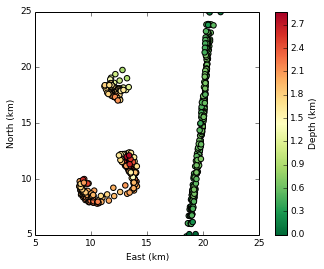In :
scene = myv.figure(size=(1000, 600))
myv.polyprisms([sill, dike], opacity=0.3, linewidth=2)
myv.points([ideal_batholith.center], color=(0, 0, 1), size=ideal_batholith.radius, opacity=0.3)
myv.points([e for e in euler_sphere.estimate_ if e > 0])
ax = myv.axes(myv.outline(bounds), ranges=[b*0.001 for b in bounds], nlabels=3, fmt='%.0f')
ax.axes.x_label, ax.axes.y_label, ax.axes.z_label = 'x', 'y', 'z'
ax.axes.font_factor = 2
myv.wall_north(bounds)
myv.wall_bottom(bounds)
scene.scene.camera.position = [-10753.852296021096, -59325.007533753123, -22266.66194804628]
scene.scene.camera.focal_point = [16722.015964135739, 13784.075401721391, 4529.0411289607291]
scene.scene.camera.view_angle = 19.2
scene.scene.camera.view_up = [0.12859903242950413, 0.29830909042254711, -0.94576634293543571]
scene.scene.camera.clipping_range = [43526.915602369831, 131500.63847030781]
scene.scene.camera.compute_view_plane_normal()
scene.scene.render()
myv.savefig('tmp/euler-solutions-3d-sphere.png')
scene.scene.camera.position = [61783.513706029873, -51534.690503972124, -18750.237814442207]
scene.scene.camera.focal_point = [14324.659515610345, 12669.434351699714, 2305.8434486142896]
scene.scene.camera.view_angle = 5.033164800000001
scene.scene.camera.view_up = [-0.14301336238889156, 0.21138117409951956, -0.96688426267807892]
scene.scene.camera.clipping_range = [41498.787075197979, 137750.00692720726]
scene.scene.camera.compute_view_plane_normal()
scene.scene.render()
myv.savefig('tmp/euler-solutions-3d-sphere-zoom.png')
myv.show()
Image(filename='tmp/euler-solutions-3d-sphere.png')

Out: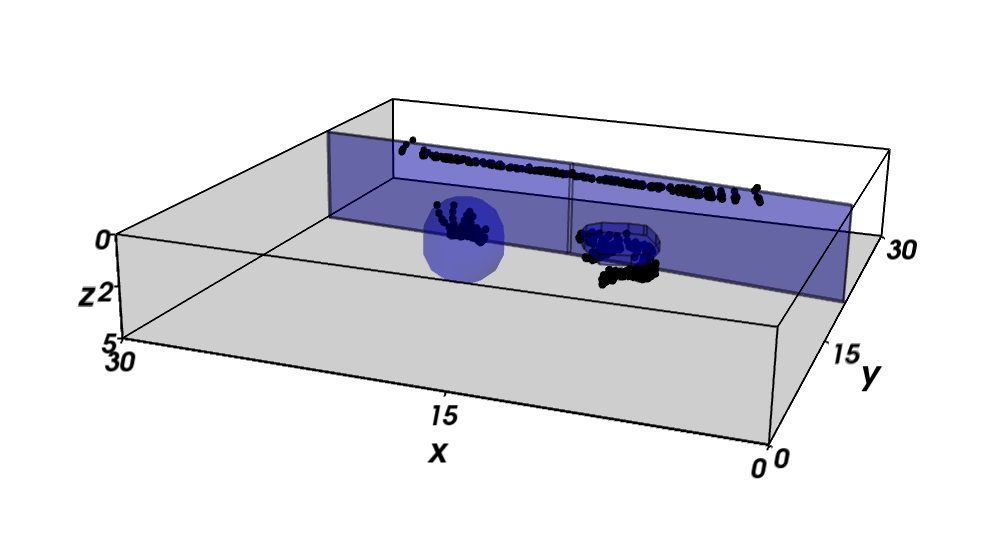In :
Image(filename='tmp/euler-solutions-3d-sphere-zoom.png')

Out: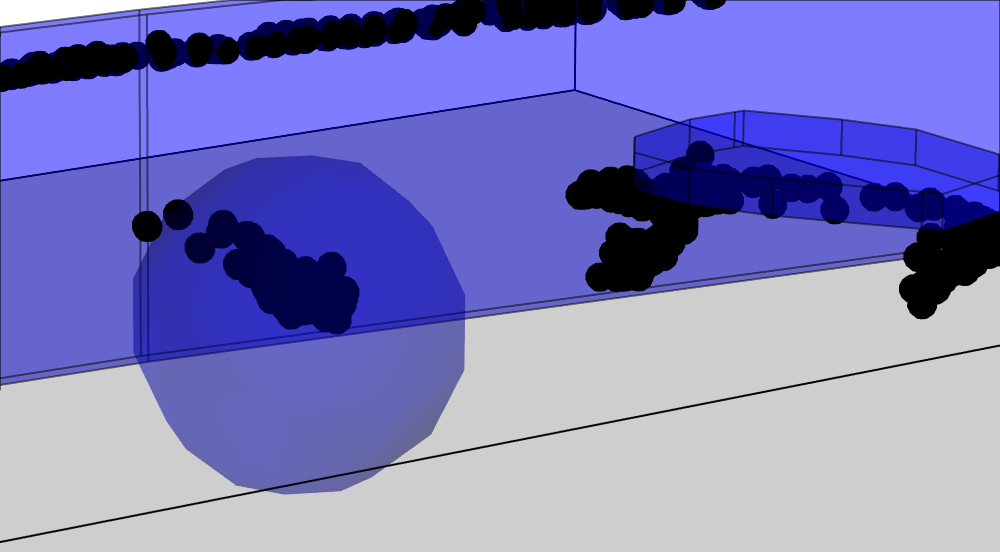Even though the solutions are closer together, there is still a spread. Notice that this happens even with an ideal source and correct structural index. What is more, the spread still has no relation with the shape of the source. Rather, it depends on the moving-window scheme and selection criterion used (Silva et al., 2001).

## Conclusions¶

The simplicity of Euler deconvolution can mislead the interpreter into thinking that the solutions are unambiguous, unbiased and represent the actual 3D form of the geologic bodies. This is not true. We have shown that the results vary greatly depending on the moving window size and structural index. Furthermore, the filtering criterion and how far the sources stray from ideal ones also influence the spread of the solutions. Thus, Euler solutions can be interpreted, at most, as an approximate location for the anomaly source. In some cases, the solutions may indicate the horizontal outline of the source.

The interpretation of a complex geological setting requires a lot of prior information. Details about the source shape can only be obtained by the introduction of harsh constraints to the ill-posed inverse problem. Euler deconvolution does not use such constraints and, thus, it may lead to wrong conclusions about the geologic source. The key concept is that the solutions must not only fit the data, but also make sense in a geological setting, a discretion that is up to the interpreter to make. Method parameters should be tunned to conform to geology, not the other way around.

In conclusion, Euler deconvolution is a powerful method for potential field interpretation. However, its results are subject to non-uniqueness and bias, as with any other geophysical inversion, and must be viewed with a critical eye. Euler deconvolution requires little prior information, computational resources, and parameter tuning. Thus, one should not expect to get the same quality of result as one would from a computationally intensive, highly regularized, 3D inversion. In short, "there is no such thing as a free lunch."

Beiki, M., and L. B. Pedersen (2010), Eigenvector analysis of gravity gradient tensor to locate geologic bodies, Geophysics, 75(6), I37–I49, doi:10.1190/1.3484098.

Reid, A. B., J. M. Allsop, H. Granser, A. J. Millett, and I. W. Somerton (1990), Magnetic interpretation in three dimensions using Euler deconvolution, Geophysics, 55(1), 80–91, doi:10.1190/1.1442774.

Silva, J. B. C., V. C. F. Barbosa, and W. E. Medeiros (2001), Scattering, symmetry, and bias analysis of source‐position estimates in Euler deconvolution and its practical implications, Geophysics, 66(4), 1149–1156, doi:10.1190/1.1487062.